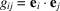International
Tables for
Crystallography
Volume D
Physical properties of crystals
Edited by A. Authier

International Tables for Crystallography (2006). Vol. D, ch. 1.1, p. 9

## Section 1.1.3.6.1. Tensor nature of the metric tensor

A. Authiera*

aInstitut de Minéralogie et de la Physique des Milieux Condensés, Bâtiment 7, 140 rue de Lourmel, 75015 Paris, France
Correspondence e-mail: aauthier@wanadoo.fr

#### 1.1.3.6.1. Tensor nature of the metric tensor

| top | pdf |

Equation (1.1.2.17)describing the behaviour of the quantitiesunder a change of basis shows that they are the components of a tensor of rank 2, the metric tensor. In the same way, equation (1.1.2.19)shows that the's transform under a change of basis like the product of two contravariant coordinates. The coefficientsandare the components of a unique tensor, in one case doubly contravariant, in the other case doubly covariant. In a general way, the Euclidean tensors (constructed in a space where one has defined the scalar product) are geometrical entities that can have covariant, contravariant or mixed components.